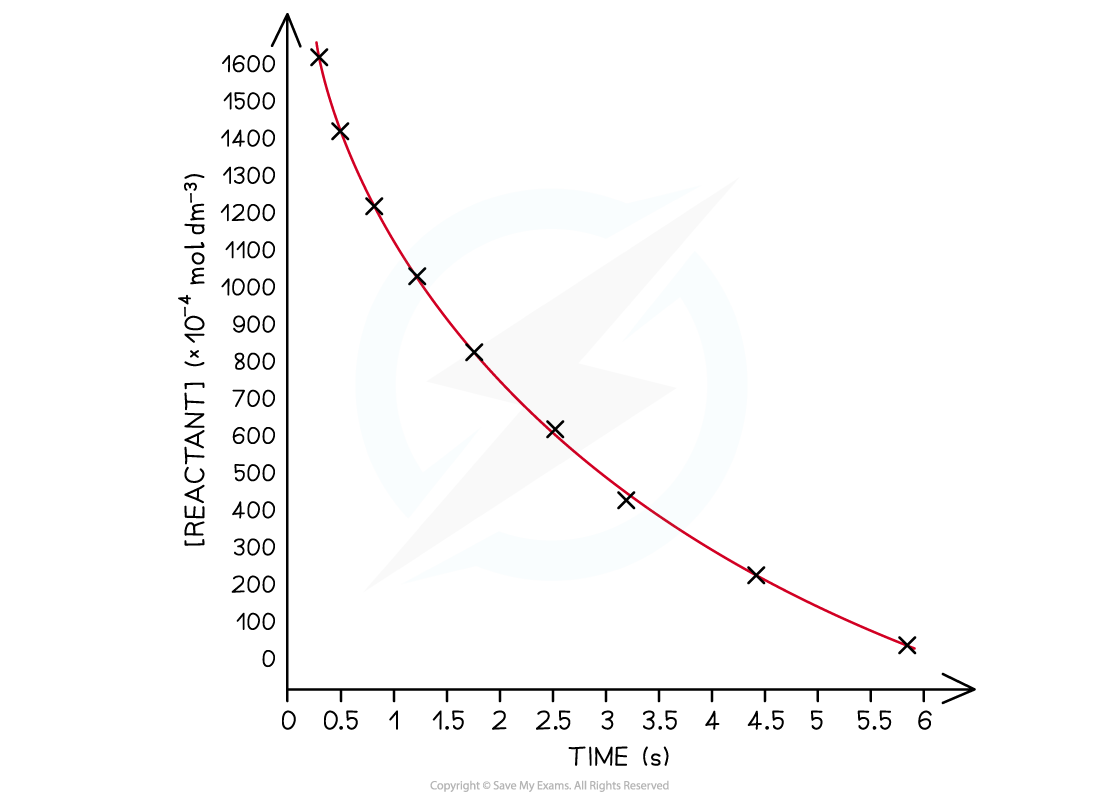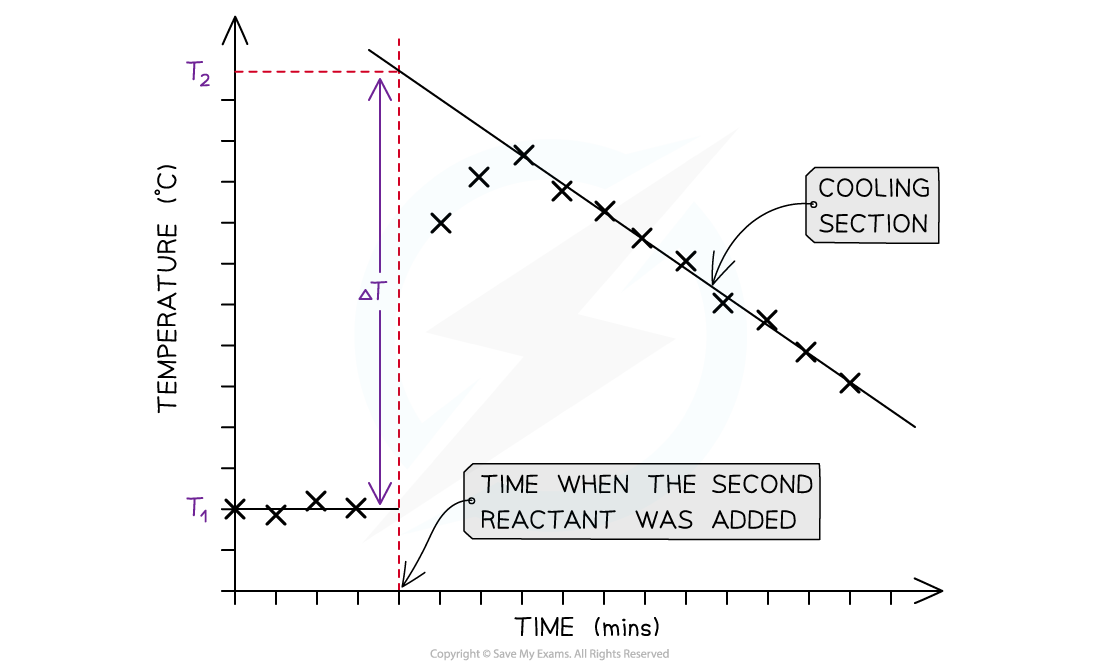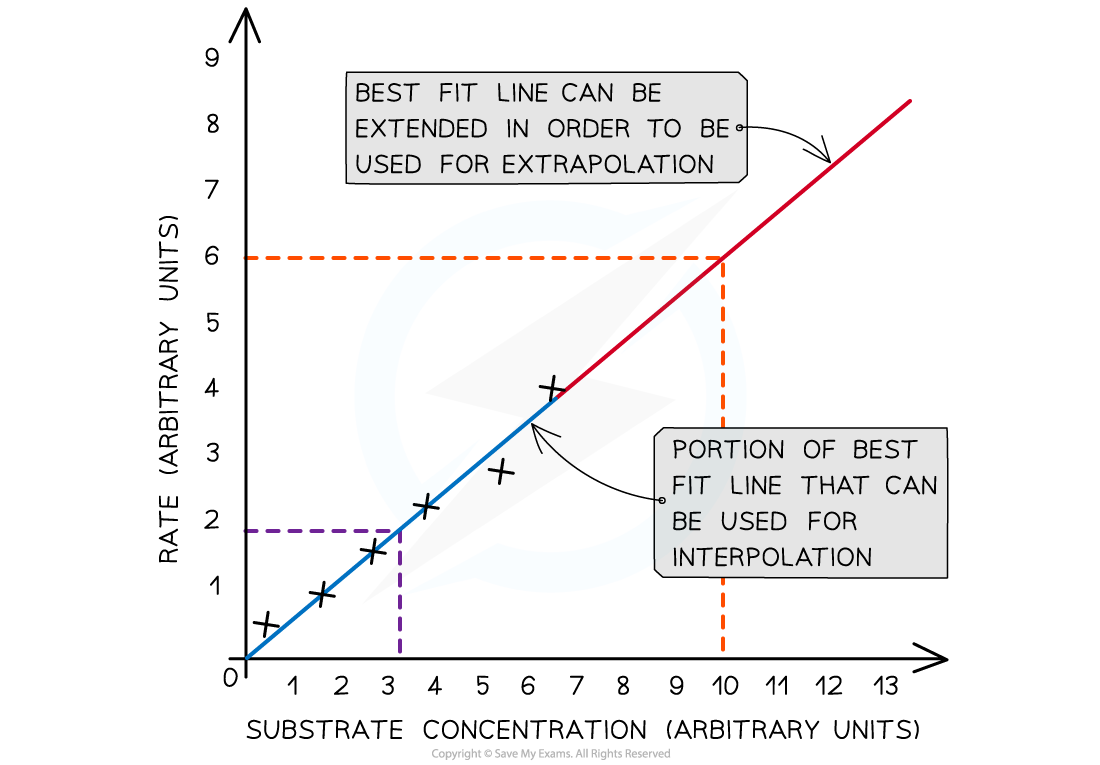# IB DP Chemistry: SL复习笔记11.2.5 Drawing Graphs

### Drawing Graphs

#### General guidance on drawing graphs

• Graphs need to have:
• Clear title
• Labelled axes
• Units on the axes
• Appropriate linear scales without any jumps
• This means the plotted graph must occupy at least half or more of the sheet or grid
• A rough rule of thumb is that if you can double the scale and still fit all the points on, then your scale is not appropriate
• Clearly shown data points
• The most common convention is to use small crosses to show the data points

Graph of concentration versus timeGraphs must shows appropriate scales, labelling and units. The independent variable usually goes on the x-axis and the dependent variable on the y-axis

• Remember the independent variable is the one you control or manipulate and the dependent variable is the one that changes as a result of your manipulation
• Always draw data points in pencil as it  makes it easier to make corrections and adjustments

### Best Fit Lines

• Students often confuse the term lines of best fit with straight lines
• Lines of best fit can be straight lines or curves (just like the example above) and:
• They show the trend of the data
• It does not have to go through all the points, but shows the general trend
• They must go through the majority of the points
• Where the data is scattered the points should be evenly distributed on either side of the best fit line
• Sometimes the best fit line has to be extended to find a value from a graph
• This is called extrapolation as this example shows from a temperature correction graph in a calorimetry investigation where the cooling section is extrapolated to find the maximum temperature rise:Extrapolation on a temperature correction graph

• Interpolation is the term used to describe the process of assuming a trend line applies between two points as this example below shows:The difference between extrapolation and interpolation on a graph

#### Exam Tip

You will have to decide if the origin, point (0,0) should be included as a data pointIf it does, it will be a good place to anchor the graph as it will be the most accurate data point